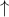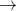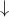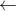# MCAT Physical : Displacement, Velocity, and Acceleration

## Example Questions

← Previous 1 3

### Example Question #1 : Displacement, Velocity, And Acceleration

A 2kg mass is suspended on a rope that wraps around a frictionless pulley attached to the ceiling with a mass of 0.01kg and a radius of 0.25m. The other end of the rope is attached to a massless suspended platform, upon which 0.5kg weights may be placed. While the system is initially at equilibrium, the rope is later cut above the weight, and the platform subsequently raised by pulling on the rope.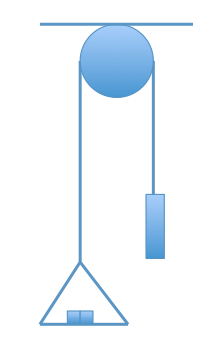If we assumed the pulley had internal friction, how would the initial and final accelerations of the platform with the individual masses change?

Initial acceleration will iecrease; final acceleration will remain the same

Initial acceleration will remain the same; final acceleration will remain the same

Initial acceleration will decrease; final acceleration will increase

Initial acceleration will decrease; final acceleration will remain the same

Initial acceleration will decrease; final acceleration will remain the same

Explanation:

When the rope is around the pulley, the acceleration is slower due to internal friction, thus the initial acceleration of the platform would be lower. Once the platform was in free fall, however, the acceleration due to gravity is constant at 9.8 m/s2; thus, the final acceleration of the platform is the same in both scenarios.

### Example Question #2 : Displacement, Velocity, And Acceleration

A runner completes a lap on a circular track in three minutes. The track has a radius of 210ft. The runner ran at a constant speed around the track.

Which of the following statements is true?

The runner's average speed is greater than the runner's average velocity

The runner's total distance traveled is equal to the runner's total displacement

The runner never accelerated while running around the track

The runner's average velocity is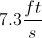The runner's average speed is greater than the runner's average velocity

Explanation:

This is a question that helps you see how displacement and velocity compare to distance and speed, respectively. Remember that the runner starts and stops at the same point on the track, so her total displacement is zero feet.

Since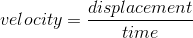, the runner's average velocity is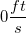. Since the runner's average speed is greater than her average velocity, we can conclude this is the correct answer. Her average speed refers to her distance, rather than displacement, and is greater than zero.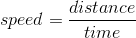This is also important to remember! Since the runner changed direction on the track, she accelerated and decelerated accordingly based on her position on the track. She did not have a constant acceleration.

### Example Question #3 : Displacement, Velocity, And Acceleration

Three blocks (in left-to-right order: block A, block B, block C) are pushed along a frictionless horizontal surface by a 50N force to the right, which acts on block A.

If the masses of the blocks are given by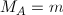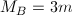, and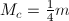, which of the following best describes the relationship between the accelerations of the blocks?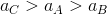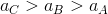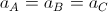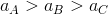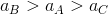Explanation:

The three blocks must remain in contact as they move, so they will each have the same velocity and acceleration regardless of their different masses. So,.

### Example Question #4 : Displacement, Velocity, And Acceleration

A man walks two kilometers north and then two kilometers west.  What is his displacement?

1. 4 kilometers
2. 4 kilometers northwest
3. 2.8 kilometers northwest
4. 8 kilometers northwest
5. none of these

1

4

2

3

5

3

Explanation:

Choice 3 is correct. Recall that physics problems tell you where to begin and end. The only thing that matters in figuring displacement is the beginning point and end point. The man effectively walked along the hypotenuse of a right triangle whose sides measured 2 km each.  Since a2 + b2 = c2, then 4 + 4 = c2, and the correct response is the square root of 8 with the direction of the displacement added for clarity.

### Example Question #5 : Displacement, Velocity, And Acceleration

A boat sails straight north at 10 knots (10 nautical miles per hour) for ten hours. As it sails, a steady wind comes from the east at 5 knots. If the helmsman makes no correction for the wind, where will the boat be compared to its starting point?

1. 50 nautical miles north of the starting point
2. 150 nautical miles north of the starting point
3. 112 nautical miles northeast of the starting point
4. 112 nautical miles northwest of the starting point
5. none of the above

5

2

3

4

1

4

Explanation:

Choice 4 is correct. In physics, it is often helpful to draw a diagram of the problem.  In this case, the respondent would draw a vector 10 hours x 10 knots from south to north (usually going towards the top of a sheet of paper) and a perpendicular vector 50 nautical miles long projecting from the arrowhead of the first vector to the left, or west. The hypotenuse of the right triangle so created is the actual path of a boat experiencing these forces. Without resorting to calculating the square root of the sum of (10,000 plus 2,500 nautical miles2), it is obvious that choice 4 is the only reasonable one.

### Example Question #6 : Displacement, Velocity, And Acceleration

A body travels 150km north, and then 500km east. Its total displacement is __________.

522km

574km

350km

650km

522km

Explanation:

To find total displacement, find the vector sum of the northern and eastern travel using the Pythagorean theorem.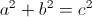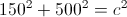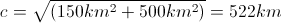Note that displacement differs from total distance traveled (which would be 650km).

### Example Question #7 : Displacement, Velocity, And Acceleration

A body moves from position X at a constant velocity, v1, for two seconds, remains at rest for three seconds, and then returns to the original position X at a constant velocity v2 after four seconds. Which of the following represents the velocity of v2?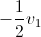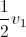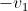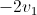Explanation:

Since it takes the body twice the time to cover the same distance, the magnitude of v2 is half of v1. Also note that the direction of the body has reversed (velocity is a vector quantity), and so v2 must be negative, with regard to v1.

v = d/t

v1 = d/2

v2 = -d/4

v2 = (-1/2)v1

### Example Question #1 : Displacement, Velocity, And Acceleration

A graph is made of the following motion by plotting time on the x-axis and velocity on the y-axis. An object accelerates uniformly between for three seconds, and then steadily increases the acceleration for the next three seconds. It remains at a constant velocity for the next two seconds. Which of the following is not true?

The slope is constant between seconds 3 and 6

The slope is constant between seconds 1 and 3

The slope is zero between seconds 6 and 8

The area under the curve is equal to displacement

The slope is constant between seconds 3 and 6

Explanation:

It is stated that between seconds 3 and 6 the object's acceleration increases. On a velocity vs. time graph, this would translate into a nonlinear curve.

Between seconds 1 and 3 the acceleration is constant, and would result in a linear velocity-time relationship. When the object travels at a constant velocity, the acceleration, which correlates to the slope, is zero.

### Example Question #1 : Displacement, Velocity, And Acceleration

For a projectile moving in a parabolic path on Earth, which of the following is true? (Ignore wind resistance.)

The acceleration of the object is zero

The net force is tangent to the path of the projectile at any given time

The net force on the object is zero

The acceleration of the object is constant

The acceleration of the object is constant

Explanation:

Acceleration is equal to the acceleration due to gravity (about 10 m/s2) and is constant while the projectile is in the air. The force on the object is given by the product of its mass and gravitational acceleration, and is also constant.

### Example Question #2 : Displacement, Velocity, And Acceleration

A particle is traveling to the right with decreasing speed. Which of the following shows the direction of acceleration?